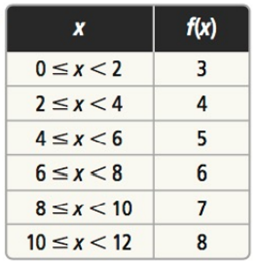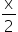Mathematics
Easy

Question

# For the following table, write a rule for f using the ceiling or floor function## Floor (x) + 1Floor () + 3 , 0 ≤ x < 12Floor () , 0 ≤ x < 12Floor (2x) + 3 , 0 ≤ x < 12Hint:

## The correct answer is: Floor () + 3 , 0 ≤ x < 12

### We have to write a rule using the floor and ceiling function. The function can be: f(x) = floor() + 3 , 0 ≤ x < 12.Recheck by putting the values of x from the table in the above equation.Hence, the correct option is C.

The ceiling function is also known as the smallest integer function. The floor function is also known as greatest integer function.

### Related Questions to study#### With Turito Foundation.#### Get an Expert Advice From Turito.